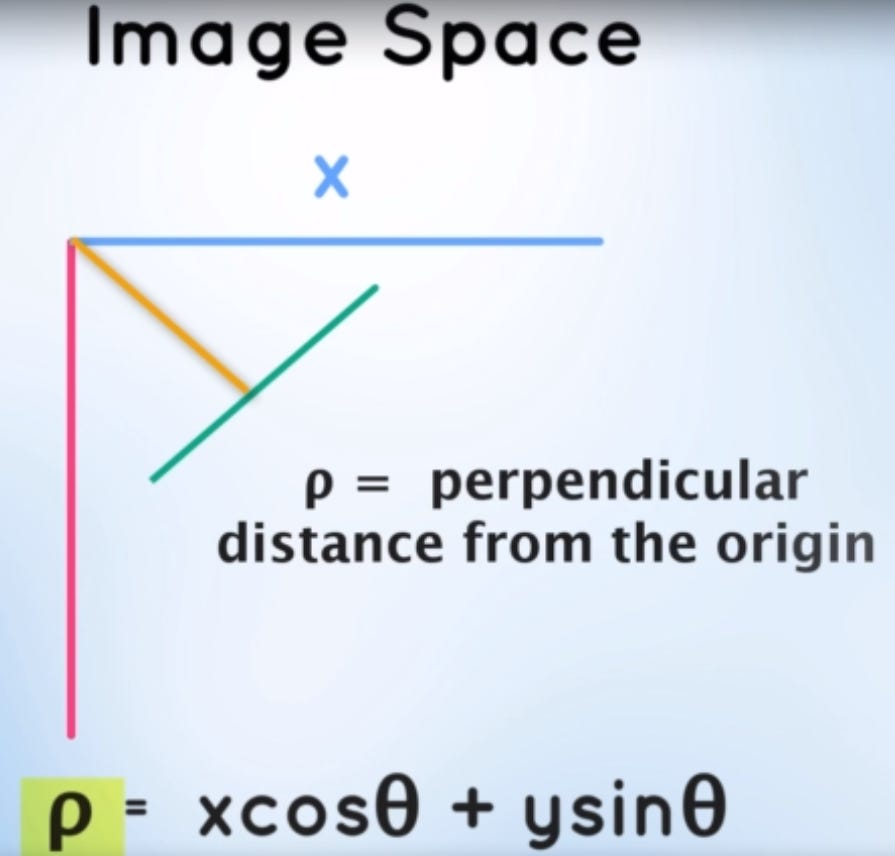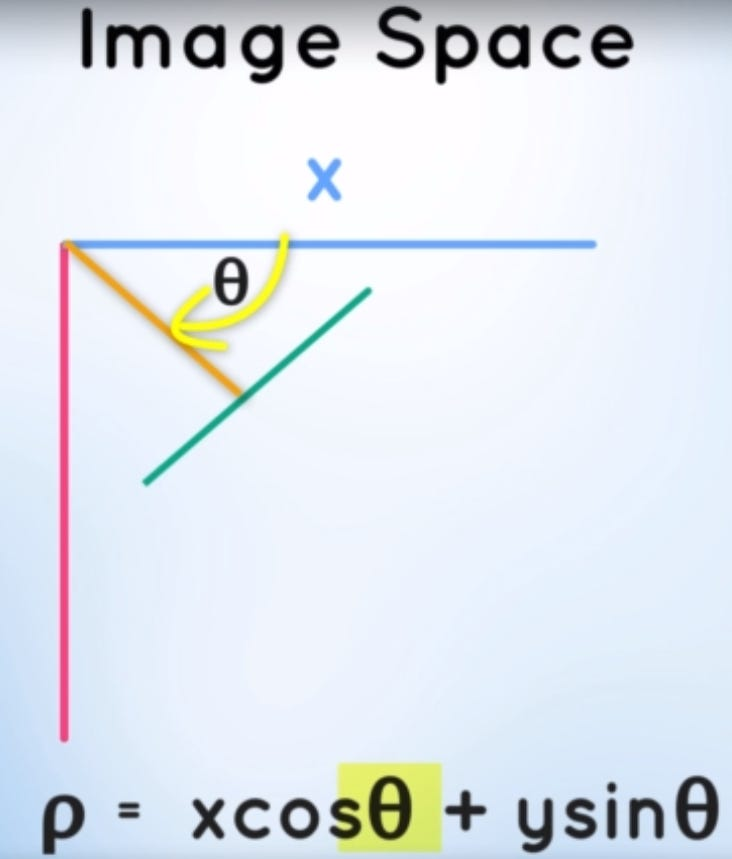# Self driving cars: From A to Z(Module 1) — 2 (Finding lane lines)

Source: Deep Learning on Medium

Welcome to Self driving car engineer module 1 part 2. I hope you liked part 1 of the series. If you haven’t looked at it you can read it here. This section will be a shorter version since we will be executing a project where in we will be detecting lane lines in a video which is nothing but a series of images. I will be explaining a step by step approach that needs to be taken in order to complete the project. The code and data set for the same will be available at my GitHub repository.

### Project Expectations

The project expects us to write code to find lanes in some of the images provided by them and then expand it to run on video files. We have learnt about finding lane lines in Part 1 of the series. In this section we will have a hands-on exercise wherein you can code alongside. I will try explaining the code step-by-step along with the mathematical details. So, lets start.

#importing some useful packages
import matplotlib.pyplot as plt
import matplotlib.image as mpimg
import numpy as np
import cv2
%matplotlib inline

First load the image and plot it to see how it looks.

plt.imshow(image)
plt.show()

Next we convert the image to gray scale and have a look at it. Notice that i have created the copy of the image and performing any processing on that image copy. Since processing the images may reflect changes in the original image which we don’t want. Converting to gray scale reduces the image dimension from 3(R,G,B) to single dimension. Detection of edges is much more accurate when done in gray scale images. Hence this conversion.

image_copy = np.copy(image)
gray = cv2.cvtColor(image_copy, cv2.COLOR_BGR2GRAY)
plt.imshow(gray, cmap = ‘gray’)
plt.show()

Step 2: Smoothing the gray scale image

Once the image has been converted to gray scale, we then perform image smoothing. Images usually contain a lot of noise which make it difficult for algorithms to detect the edges. Smoothing the images does the detection task easier for us.

blur = cv2.GaussianBlur(gray,(5,5), 0)
plt.imshow(blur, cmap = ‘gray’)
plt.show()

You would be thinking what the (5,5) means in the first line of the code. Let me explain what image smoothing means.

The Gaussian blur performs smoothing by averaging out the pixel intensity values through a weighted average kernel. (5,5) is the kernel size that is used to perform the smoothing operation. You are free to try out with different kernel size.

Step 3: Detecting edges using canny edge detection algorithm

The purpose of edge detection in general is to significantly reduce the amount of data in an image, while preserving the structural properties to be used for further image processing.

The algorithm runs in 5 separate steps:

1. Smoothing: Blurring of the image to remove noise.
2. Finding gradients: The edges should be marked where the gradients of the image has large magnitudes.
3. Non-maximum suppression: Only local maxima should be marked as edges.
4. Double thresholding : Potential edges are determined by thresholding.
5. Edge tracking by hysteresis: Final edges are determined by suppressing all edges that are not connected to a very certain (strong) edge.

For details of each of the steps you can visit here

But when it comes to the code it just requires a single line of code to be executed as shown below:

low_threshold = 150
high_threshold = 300
canny = cv2.Canny(blur, low_threshold, high_threshold)

Step 4 : Creating masked image using region of interest

Next we create an image mask which is a black image having same dimension as that of the original image. We then take the original image and identify the region of interest for us. In this case we have a triangle in place for the ROI which is then superimposed on the mask using opencv fillpoly() function and a region of interest mask is generated.

imshape = image.shape
vertices = np.array([[(400, imshape), (520, 378), (600, imshape)]])

Now we have the ROI mask and the canny image as well. The next step is to perform a bitwise and operator between both the images to get the Region of interest canny image.

The bit wise and operator looks like 00000000 in the black section and 11111111 in the white section of the above image. When a bitwise_and operation is performed between the values and the values in the canny image the and operation with 00000000 will always generate a 00000000 that is a black pixel in the resulting image whereas a 11111111 will not have any effect on the resulting image and will generate a similar structure as the of the canny image in the white section.

00000000 & 10110000 = 00000000

11111111 & 10110000 = 10110000

Hence the resulting image of applying the bit wise and operator is shown below.

Step 5 : Applying Hough transform on the image

Before applying the hough transform method let us go through some of the theory behind it. The images in the image space is represented by the usual x and y axis as a 2D matrix of rows and columns that represents dimension of the image. The image in image space is represented by y = mx + b if we plot m and b as separate parameters along the x and y axis, this is called the parameter or hough space.

Also a line in the image space can be represented by a point in the hough space. e.g. we know in the above image the y intercept is 2 and the slope is 3. hence if we plot this line in the hough space it can be represented as below.

Similarly a point in the image space is represented by a line in the hough space since many lines can possibly pass through a single point in the image space which can take more than 1 value in of m and b. Similarly we have two points in the image space it can be represented by two lines in the hough space. And the line that is consistent with the two points and passes through both the points in the image space is the point in the hough space.

You might be thinking why is this relevant ? Well this idea of identifying possible lines from a series of points is how we are going to find lines in our gradient image. The gradient image is just a series of point which represent edges in our image space.

We can clearly say that is series of point mentioned in yellow in the above image is a line. If we represent this series of points in the image space into multiple lines in the hough space and ask you that the points in the image space represents a line and what is that line? We can see multiple intersections of the lines in the hough space since there is no single line that pass through all the point in the image space. In order to solve this problem we will divide the hough space into grid cells and we can notice that all the intersection of lines fall in a common bin inside our grid. For every point of intersection we are going to cast votes inside the bin it belongs to. The bin with the maximum number of votes is going to be out line

The b and m values for the bin will be the slope and intercept of the line in the image space. Now you know how the lines and points can be interpreted in the image and hough space and vice versa. But there is still a problem going forward with this method. If the line in the image space is vertical then the slope or the m values becomes infinity which cannot be represented in hough space. So we need to have a much robust representation of lines where we do not encounter any numeric problem as we are encountering now. To overcome this, we represent our lines in polar coordinates (rho and theta). The equation of a line in polar coordinates is

rho = x*cos(theta) + ysin*(theta)rho in polar coordinates is the vertical distance of line from originTheta indicates angle of inclination of the line from the X-axis which is measured in radians with positive X-axis

Now suppose we take a point (5, 2) in polar coordinates a compute rho and theta for the following line

But how will a point in the image space with polar coordinates be represented in the hough space.

It will be represented as a sin curve in the hough space. All the possible values that the point can take to represent a line line above can be represented as the sin curve in the hough space as seen above. Sililarly if we have series of point the the image space it can be represented as shown below.

Now we are ready to code.

`# Define the Hough transform parameters# Make a blank the same size as our image to draw onrho = 1 # distance resolution in pixels of the Hough gridtheta = np.pi/180 # angular resolution in radians of the Hough gridthreshold = 2     # minimum number of votes (intersections in Hough grid cell)min_line_length = 4 #minimum number of pixels making up a linemax_line_gap = 5    # maximum gap in pixels between connectable line segmentsline_image = np.copy(image)*0 # creating a blank to draw lines on# Run Hough on edge detected image# Output "lines" is an array containing endpoints of detected line segmentslines = cv2.HoughLinesP(masked_edges, rho, theta, threshold, np.array([]), min_line_length, max_line_gap)`

The Houghlines() function generate a series of (x1, y1)(x2, y2) values which needs to be plotted together inorder to produce the lane line lines in the masked image.

`# Iterate over the output "lines" and draw lines on a blank imagefor line in lines:    for x1,y1,x2,y2 in line:        cv2.line(line_image,(x1,y1),(x2,y2),(255,0,0),10)`

Now we can add this lines to our original image and see how it looks like.

line_canny = cv2.addWeighted(image_copy, 0.8, line_image, 1, 0)
plt.imshow(line_canny)
plt.show()

Finally we are done with the image section. How you got an idea of how an image needs to be processed along with the computations happening in the background. Now we will extend this to videos. We will begin by importing the required packages for video analysis and processing in python

from moviepy.editor import VideoFileClip
from IPython.display import HTML

clip1 = VideoFileClip(“solidWhiteRight.mp4”)
white_clip = clip1.fl_image(process_image) #NOTE: this function expects color images!!
white_clip.write_videofile(white_output, audio=False)

The videofileclip() function reads in as videofile and make it ready for analysis and processing. The fl_image() allows us to perform preprocessing by taking each frame of video as an image and applying each processing step as we did for our image. The preprocess is a function that i wrote which consists of each of the step that we performed during preprocessing. And finally the write_videofile() saves the output of our processing on each image and merging it together to form a video. This is how the resulting video looks like:

This was the end of our project and we were successfully able to find lane lines in videos as well. Next up will be the base of all the AI problems that we will learn which are neural networks. We will learn the theory behind it and code a Neural Network from scratch and understand the mathematical intuition behind it.

References:

https://www.udemy.com/applied-deep-learningtm-the-complete-self-driving-car-course